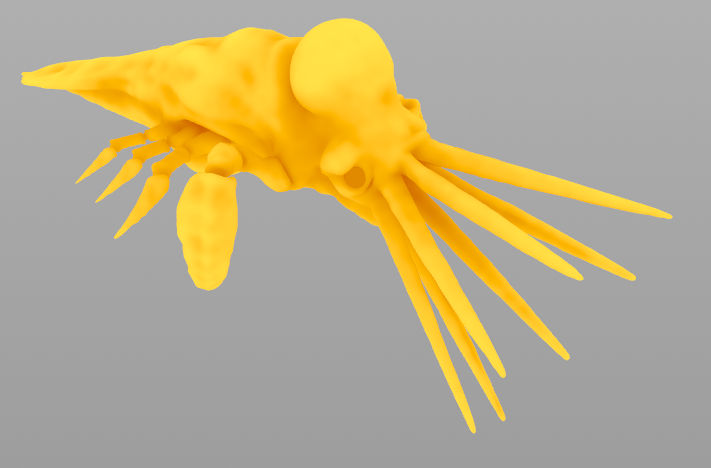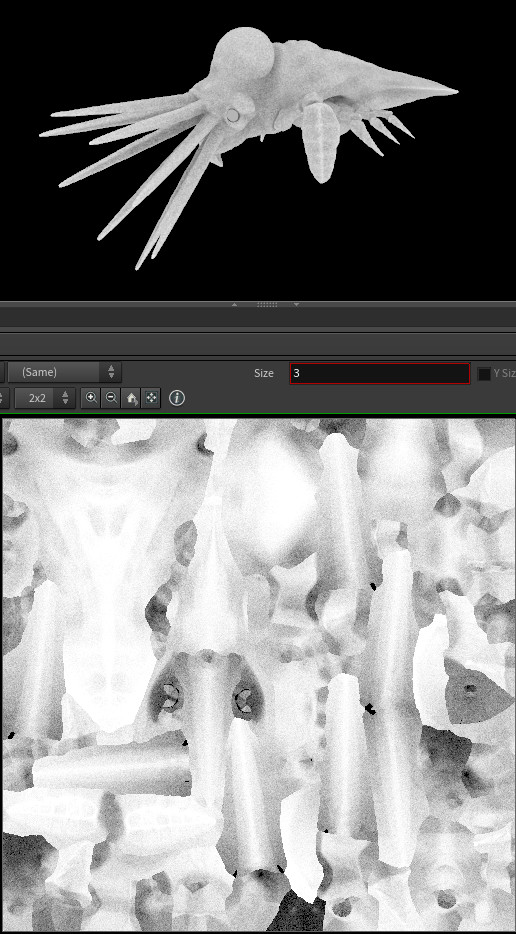# Ambient Occlusion texturing

calendar_today

04.05.2022

label

VEX, Texturing

mouse

Houdini 18.0

### 1 Introduction

Two methods for generating occlusion texture maps. The first one uses raycasting on a per texture pixel basis. The second method uses raycasting and filtering from a scattered point cloud on the mesh surface.

### 2 Per-texel raycasting in COPsA minimalist generator for creating occlusion textures right in COPs.

``````// INPUT
vector pos_tex = set(X, Y, 0.0);
vector pos_mesh = uvsample(geo_mesh, 'P', 'uv', pos_tex);
vector nml_mesh = uvsample(geo_mesh, 'N', 'uv', pos_tex);

// OCCLUSION
float occ_sum = 0.0;
for(int i = 0; i < samples; i++){
vector2 u = rand(pos_tex * i);
vector dir = sample_direction_cone(nml_mesh, a, u);
vector pos_ray = pos_mesh + nml_mesh * 1e-3;
vector dir_ray = dir * range;
vector pos_hit;
vector uvw_hit;
int prim_hit = intersect(geo_mesh, pos_ray, dir_ray, pos_hit, uvw_hit);
occ_sum += prim_hit == -1;
}
float occ = occ_sum / float(samples);

// OUTPUT
vector color = vector(occ);
assign(R, G, B, color);``````

### 3 Point cloud based raycastingA softer and more efficient point cloud based approach. Scattered points on the mesh surface are used for raycasting the occlusion. The second wrangle smoothly interpolates the result across the texture.

``````vector pos_ray = v@pos + v@nml * 1e-5;

float occ_sum = 0.0;
for(int i = 0; i < samples; i++){
vector2 seed = rand(v@pos + i);
vector dir_ray = sample_hemisphere(v@nml, bias, seed) * range;
vector pos_hit;
vector uvw_hit;
int prim_hit = intersect(geo_mesh, pos_ray, dir_ray, pos_hit, uvw_hit);
occ_sum += prim_hit != -1;
}

f@occ = 1.0 - (occ_sum / float(samples));
assign(R,G,B, f@occ);``````
``````int handle = pcopen(geo_pc, 'P', v@pos, range, samples);
vector color = pcfilter(handle, 'Cd');

f@occ = color.x;
assign(R,G,B, color);``````